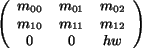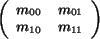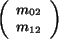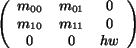## CGAL::Aff_transformation_2<Kernel>

### Definition

The class Aff_transformation_2<Kernel> represents two-dimensional affine transformations. The general form of an affine transformation is based on a homogeneous representation of points. Thereby all transformations can be realized by matrix multiplications.

Multiplying the transformation matrix by a scalar does not change the represented transformation. Therefore, any transformation represented by a matrix with rational entries can be represented by a transformation matrix with integer entries as well. (Multiply the matrix with the common denominator of the rational entries.) Hence, it is sufficient to use the number type Kernel::RT to represent the entries of the transformation matrix.

CGAL offers several specialized affine transformations. Different constructors are provided to create them. They are parameterized with a symbolic name to denote the transformation type, followed by additional parameters. The symbolic name tags solve ambiguities in the function overloading and they make the code more readable, i.e., what type of transformation is created.

Since two-dimensional points have three homogeneous coordinates, we have a 3 × 3 matrix (mij)i,j=0...2.

If the homogeneous representations are normalized (the homogenizing coordinate is 1), then the upper left 2 × 2 matrix realizes linear transformations. In the matrix form of a translation, the translation vector (v0,v1,1) appears in the last column of the matrix. The entries m20 and m21 are always zero and therefore do not appear in the constructors.

### Creation

Aff_transformation_2<Kernel> t ( Identity_transformation);
introduces an identity transformation.

Aff_transformation_2<Kernel> t ( const Translation, Vector_2<Kernel> v);
introduces a translation by a vector v.

Aff_transformation_2<Kernel> t ( const Rotation, Direction_2<Kernel> d, Kernel::RT num, Kernel::RT den = RT(1));
approximates the rotation over the angle indicated by direction d, such that the differences between the sines and cosines of the rotation given by d and the approximating rotation are at most num/den each.
 Precondition: num/den>0 and d != 0.

Aff_transformation_2<Kernel> t ( const Rotation, Kernel::RT sine_rho, Kernel::RT cosine_rho, Kernel::RT hw = RT(1));
introduces a rotation by the angle rho.
 Precondition: sine_rho2 + cosine_rho2 == hw2.

Aff_transformation_2<Kernel> t ( const Scaling, Kernel::RT s, Kernel::RT hw = RT(1));
introduces a scaling by a scale factor s/hw.

 Aff_transformation_2 t ( Kernel::RT m00, Kernel::RT m01, Kernel::RT m02, Kernel::RT m10, Kernel::RT m11, Kernel::RT m12, Kernel::RT hw = RT(1));
introduces a general affine transformation in the 3x3 matrix. The sub-matrix hw-1contains the scaling and rotation information, the vector hw-1contains the translational part of the transformation.

 Aff_transformation_2 t ( Kernel::RT m00, Kernel::RT m01, Kernel::RT m10, Kernel::RT m11, Kernel::RT hw = RT(1)); introduces a general linear transformation, i.e. there is no translational part.

### Operations

The main thing to do with transformations is to apply them on geometric objects. Each class Class_2<Kernel> representing a geometric object has a member function:

Class_2<Kernel> transform(Aff_transformation_2<Kernel> t).

The transformation classes provide a member function transform() for points, vectors, directions, and lines:

 Point_2 t.transform ( Point_2 p) Vector_2 t.transform ( Vector_2 p) Direction_2 t.transform ( Direction_2 p) Line_2 t.transform ( Line_2 p)

CGAL provides function operators for these member functions:

 Point_2 t.operator() ( Point_2 p) Vector_2 t.operator() ( Vector_2 p) Direction_2 t.operator() ( Direction_2 p) Line_2 t.operator() ( Line_2 p)

### Miscellaneous

 Aff_transformation_2 t.operator* ( s) composes two affine transformations. Aff_transformation_2 t.inverse () gives the inverse transformation. bool t.is_even () returns true, if the transformation is not reflecting, i.e. the determinant of the involved linear transformation is non-negative. bool t.is_odd () returns true, if the transformation is reflecting.

The matrix entries of a matrix representation of a Aff_transformation_2<Kernel> can be accessed trough the following member functions:

 Kernel::FT t.cartesian ( int i, int j) Kernel::FT t.m ( int i, int j) returns entry mij in a matrix representation in which m22 is 1. Kernel::RT t.homogeneous ( int i, int j) Kernel::RT t.hm ( int i, int j) returns entry mij in some fixed matrix representation.

For affine transformations no I/O operators are defined.

Identity_transformation, Rotation, Scaling, Translation
rational_rotation_approximation

### Example

```  typedef Cartesian<double>        K;
typedef Aff_transformation_2<K>  Transformation;
typedef Point_2<K>               Point;
typedef Vector_2<K>              Vector;
typedef Direction_2<K>           Direction;

Transformation rotate(ROTATION, sin(pi), cos(pi));
Transformation rational_rotate(ROTATION,Direction(1,1), 1, 100);
Transformation translate(TRANSLATION, Vector(-2, 0));
Transformation scale(SCALING, 3);

Point q(0, 1);
q = rational_rotate(q);

Point p(1, 1);

p = rotate(p);

p = translate(p);

p = scale(p);
```

The same would have been achieved with

```
Transformation transform = scale * (translate * rotate);
p = transform(Point(1.0, 1.0));
```